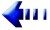Hilbert's Tenth Problem

Statement of the problem
(in English)

10. Determination of the solvability of a Diophantine equation. Given a diophantine equation with any number of unknown quantities and with rational integral numerical coefficients: To devise a process according to which it can be determined by a finite number of operations whether the equation is solvable in rational integers.main page Statement's translation: German English Russian French also Chinese

Laboratory of Mathematical Logic at
St. Petersburg Division of Steklov Institute of Mathematics (POMI) of